# Class 12 Physics: CBSE Sample Question Paper- Term I (2021-22)- 3 Notes | Study Physics Class 12 - NEET

## NEET: Class 12 Physics: CBSE Sample Question Paper- Term I (2021-22)- 3 Notes | Study Physics Class 12 - NEET

The document Class 12 Physics: CBSE Sample Question Paper- Term I (2021-22)- 3 Notes | Study Physics Class 12 - NEET is a part of the NEET Course Physics Class 12.
All you need of NEET at this link: NEET

Class-XII
Time: 90 Minutes
Max. Marks: 35

General Instructions :

1. The Question Paper contains three sections.
2. Section A has 25 questions. Attempt any 20 questions.
3. Section B has 24 questions. Attempt any 20 questions.
4. Section C has 6 questions. Attempt any 5 questions.
5. All questions carry equal marks.
6. There is no negative marking.
Section - A

This section consists of 25 multiple choice questions with overall choice to attempt any 20 questions. In case more than desirable number of questions are attempted, ONLY first 20 will be considered for evaluation.

Q.1: When an uncharged conducting sphere is brought near a charged conducting sphere, how the charge on conducting sphere be affected?
(a) increased
(b) decreased
(c) remain same
(d) zero

Charge on conductor B remains same and it induces equal and opposite charge on uncharged conducting sphere.

Q.2: On-going away from a point charge as well as dipole, electric field due to dipole decreases with the ___________ rate as compare to point charge?
(a) same
(b) rapid
(c) slow
(d) None of these

Electric field due to point charge E ∝ 1/r2 while, electric field due to dipole is E ∝ 1/r3. This clearly indicates that electric field due to dipole decreases more rapidly.

Q.3: Two metallic spheres of radii 1 cm and 3 cm are given charges of –1 × 10–2 C and 5 × 10–2 C, respectively. If these are connected by a conducting wire, the final charge on the bigger sphere is :
(a) 3 × 10–2 C
(b) 4 × 10–2 C
(c) 1 × 10–2 C
(d) 2 × 10–2 C

After connecting with the wire, they have equal potential
So, V1 = V2
KQ1/3 = KQ1/1, Hence, Q1 is the charge of sphere having radius 3 cm.
So, Q1 = 3Q2
When they are connected, Q1 + Q2 = 4 × 10–2 C
So, Q2 = 1 × 10–2C and Q= 3 × 10–2 C

Q.4: Which one of the following does not obey inverse square law?
(a) electric field due to a point charge
(b) electric potential due to a dipole
(c) electric potential due to a point charge
(d) electric force between two charged particles.

• Electric field due to a point charge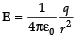• Electric potential due to a dipole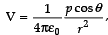where p = magnitude of dipole moment.
• Electric potential due to a point charge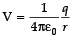.
• From Coulomb’s law, Force between two charged particles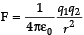.

Q.5: An electron and a proton are accelerated with the same potential. Which one of them higher velocity
(a) electron
(b) proton
(c) both have same velocity
(d) velocity will be zero

Since both the proton and electron are accelerated through the same potential, work done by an electric field will be same. This work done is equal to the kinetic energy, which is also the same for electron and proton.
⇒ 1/2mv2 = eV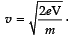Since potential is same for electron and proton.
Therefore, v ∝ √1/m
As the mass of an electron is less than the mass of a proton, therefore electron will acquire a higher velocity.

Q.6: Dielectric constant in terms of capacitance of capacitor
(a)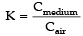(b)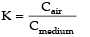(c)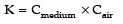(d)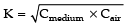If Cmedium is the value of the capacitance of a capacitor in a medium and Cair is the capacitance, the dielectric constant K is defined as.

Q.7: When the plates of a charged parallel-plate capacitor are brought closer, the potential difference between the plates
(a) decreases
(b) increases
(c) remain same
(d) becomes zero

The capacitance of a parallel plate capacitor is inversely proportional to the distance between the plates i.e. C ∝ 1 / d . So, on decreasing d, C will increase. Since C is inversely proportional to V as C = Q/V. Therefore, V will decrease.

Q.8: Three concentric spherical shells have radii a, b and c (a < b < c) and have surface charge densities  σ, – σ and σ respectively. If VA, VB and Vdenote the potentials of the three shells, then for c = a + b, we have :
(a) VC = VB ≠ VA
(b) VC ≠ VB ≠ VA
(c) VC = VB = VA
(d) VA = V≠ V
B

Given c = a + b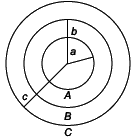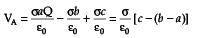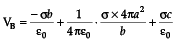=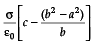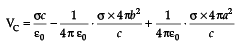=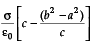=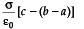VA =VC≠ VB

Q.9: When Ohm’s law is not obeyed in a conductor
(a) if temperature changes
(b) if conductor is under any strain
(c) both (A) and (B)
(d) none of these.

Ohms law is defined at the constant temperature, which states that current flowing through a conductor is directly proportional to the applied potential difference across the conductor. Additionally, if conductor is under any strain, the conductor becomes nonohmic and graph between V and I may not be straight.

Q.10: Specific resistance of a wire is defined as
(a) L/RA
(b) RA/L
(c) A/RL
(d) RL/A

Specific resistance ρ is defined as the resistance R offered per unit length L and unit cross-sectional area A i.e. ρ =  RA / L

Q.11: In any network of wires carrying current, the algebraic sum of all current at node is equal to
(a) sum of all incoming currents
(b) sum of all outgoing currents
(c) zero
(d) infinite

Kirchhoff’s first law states that the sum of incoming current at any node in a circuit is equal to the sum of outgoing current. Therefore, the algebraic sum of all current meeting at a node must equal zero.

Q.12: A bar magnet is cut into two equal parts by a plane parallel to the magnetic axis. Which physical quantities remain unchanged?
(a) magnetic moment
(b) pole strength
(c) intensity of magnetization
(d) none of these

Since, pole strength, magnetic moment and moment of inertia are the properties, which depend on the dimension of the body whereas intensity of magnetization depends on the nature of the material. therefore, by cutting a bar magnet into two parts, intensity of magnetization remains same.

Q.13: Direction of magnetic dipole moment
(a) north to south
(b) south to north
(c) east to west
(d) west to east

Magnetic dipole moment is a vector quantity and its direction is from south pole to north pole.

Q.14: A moving conductor coil produced an induced e.m.f., this statement is according to which law
(a) Coulomb’s law
(c) Lenz’s law
(d) Ampere’s law

Faraday’s law states that when the magnetic flux linking a circuit change, an electromotive force is induced in the circuit, which is proportional to the rate of change of the flux. Therefore, moving coil produces induced emf.

Q.15: A cylindrical bar magnet is kept along the axis of circular coil. On rotating a magnet about its axis, the coil will have induced
(a) a current
(b) an e.m.f.
(c) both (A) and (B)
(d) no current

When a cylindrical bar magnetic is rotated about its axis, the lines of force remain same, resulting in no change in the flux through a coil. Thus, there is no current induced in a coil.

Q.16: Production of induced e.m.f. in a coil due to the change of current in the same coil is
(a) self-induction
(b) mutual-induction
(c) dynamo
(d) none of these

When a current is changed in a coil, the magnetic flux is also changed. This flux is due to its own current. Therefore, the property is accordance with the self-induction.

Q.17: Direction of induced current in a wire in a magnetic field is given by
(a) Fleming’s left-hand rule
(b) Fleming’s right-hand rule
(c) right-hand clasp rule
(d) Ampere’s rule

Direction of induced current in a wire in a magnetic field is given by Fleming’s right-hand rule, which stats that if fore finger, middle finger and thump of our right hand are extended perpendicular to each other, then

• fore finger is pointed towards the direction of magnetic field.
• middle finger is pointed towards the direction of induced current.
• thump is pointed towards the direction of motion or applied force.

Q.18: There is an electric field E in X-direction. If the work done on moving a charge 0.2 C through a distance of 2 m along a line making an angle 60° with the X-axis is 4.0, what is the value of E :
(a) √3 N/C
(b) 4 N/C
(c) 5 N/C
(d) 20 N/C

Work done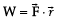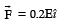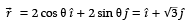Now W = 0.2E =4
E = 20 N/C

Q.19: Magnetic needle is kept in a non-uniform magnetic field experience
(a) a force as well as a torque
(b) a torque but not a force
(c) a force and a torque
(d) a force but not a torque

Field being non-uniform, the poles of the needle will experience non-uniform forces. Hence, the needle experiences a force as well as a torque.

Q.20: A ferromagnetic substance is heated above its curie temperature. Which of the following statements is correct?
(a) Ferromagnetic domains get perfectly arranged.
(b) Ferromagnetic domains get randomly arranged.
(c) Ferromagnetic domains are not at all influenced.
(d) Ferromagnetic material transforms into diamagnetic substance.

On heating above Curie temperature, Ferromagnetic domains get randomly arranged and it transforms into paramagnetic substance.

Q.21: ABC is an equilateral triangle. Charges +q are placed at each corner. The electric intensity at O will be :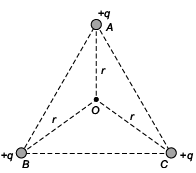(A) 1/4πε0 × q/r2
(B) 1/4πε0 × q/r
(C) Zero
(D) 1/4πε0 × 3q/r2

Since, all charges are equal in magnitude and equidistant from point O then magnitude of electric field intensity will be equal. Electric field intensity is vector quantity. So direction of vectors of all three will be 120° apart from each other, so by adding these three vectors we get zero.

Q.22: Three capacitors are connected to D.C. source of 100 volts shown in the adjoining figure. If the charge accumulated on plates of C1, C2 and C3 are qa, qb, qc, qd, qe and qf respectively, then :
(A) qb + q+ qf = 100/9 C
(B) qb + qd + qf = 0
(C) qa + qc + qe=50 C
(D) qb = qd = qf

In series combination, charge is same on each capacitor, hence qb = qd = qf

Q.23: Power factor of the ac circuit varies between
(a) –1 and 0
(b) 0 to 1
(c) –1 and +1
(d) none of these

Power factor of the ac circuit is given by
cos ∅ = R/Z
=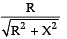When circuit contains only resistance, then
Z = R
∴ cos ∅ = 1
When circuit contains only inductance then
R = 0

∴ cos ∅ = 0

Q.24: Two different wire loops are concentric and lie in the same plane. The current in the outer loop is clockwise and increase with time. The induced current in the inner loop is
(a) anti-clockwise
(b) clockwise
(c) zero
(d) in a direction depending on the ratio of loop radii

Lenz’s law states that the induced current opposes the change in magnetic flux. So, the current in inner loop will be in opposite direction i.e. anti-clockwise.

Q.25: The r.m.s.value of potential difference V in the following figure is
(a) V0
(b) V0/2
(c) V0/√2
(d) V0/4

Given that
V =  V0 for 0 ≤ t ≤ T/2
= 0 for T/2 ≤ t ≤ T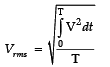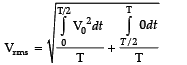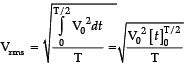Section - B
This section consists of 24 multiple choice questions with overall choice to attempt any 20 questions. In case more than desirable number of questions are attempted, ONLY first 20 will be considered for evaluation.

Q.26: A cylinder of radius r and length l is placed in an uniform electric field parallel to the axis of the cylinder. The total flux for the surface of the cylinder is given by:
(a) zero
(b) π r2
(c) E πr2
(d) 2 Eπ r
2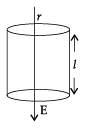Since – ve electric flux. = +ve flux electric flux enclosed with a cylinder here
∴ Total electric flux = 0.

Q.27: Two parallel large thin metal sheets have equal surface densities 26.4 × 10–12 C/m2 of opposite signs. The electric field between these sheets is:
(a) 1.5 N/C
(b) 1.5 × 10–16 N/C
(c) 3 × 10–10 N/C
(d) 3 N/C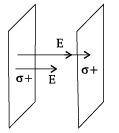Surface charge density,
σ = 26.4 ×10−12 C/m2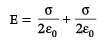=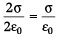=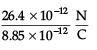=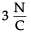Q.28: Consider an uncharged conducting sphere. A positive point charge is placed outside the sphere. The net charge on the sphere is then:
(a) negative and uniformly distributed over the surface of sphere.
(b) positive and uniformly distributed over the surface of sphere.
(c) negative and appears at a point the surface of sphere closest to point charge.
(d) zero.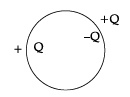Equal and opposite charges appear on the nearby conductor due to induction, but still net charge on the conductor is zero. Correct option (D).

Q.29: Three Charges 2q, – q and – q lie at vertices of a triangle. The value of E and V at centroid of triangle will be:
(a) E ≠ 0 and V ≠ 0
(b) E = 0 and V = 0
(c) E ≠ 0 and V = 0
(d) E = 0 and V ≠ 0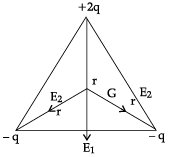Net Electric field intensity at G ≠ O Net Potential at G,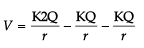= 0

Q.30: Two parallel plate capacitors X and Y, have the same area of plates and same separation between plates. X has air and Y with dielectric of constant 2 , between its plates. They are connected in series to a battery of 12 V. The ratio of electrostatic energy stored in X and Y is:
(A) 4 : 1
(B) 1 : 4
(C) 2 : 1
(D) 1 : 2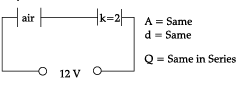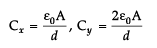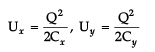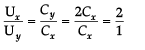Q.31: Which among the following, is not a cause for power loss in a transformer:
(a) Eddy currents are produced in the soft iron core of a transformer.
(b) Electric flux sharing is not properly done in primary and secondary coils.
(c) Humming sound produced in transform due to magnetostriction.
(d) Primary coil is made up of a very thick copper wire.

Correct statement is option (D) as primary coil made of thick copper wire has very less R. Therefore negligible power loss. Rest all options are reasons for power losses in a transformer.

Q.32: An alternating voltage source of variable angular frequency ‘w’ and fixed amplitude ‘V’ is connected in series with a capacitance C and electric bulb of resistance R (inductance zero). When ‘w’ is increased:
(a) The bulb glows dimmer.
(b) The bulb glows brighter.
(c) Net impedance of the circuit remains unchanged.
(d) Total impedance of the circuit increases.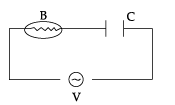w↑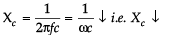I ↑ ∴  Brightness of the bulb will ↑.

Q.33: A solid spherical conductor has charge +Q and radius R. It is surrounded by a solid spherical shell with charge -Q, inner radius 2R, and outer radius 3R. Which of the following statements is true?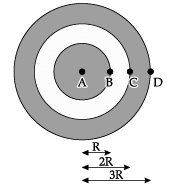(a) The electric potential has a maximum magnitude at C and the electric field has a maximum magnitude at A.
(b) The electric potential has a maximum magnitude at D and the electric field has a maximum magnitude at B.
(c) The electric potential at A is zero and the electric field has a maximum magnitude at D.
(d) Both the electric potential and electric field achieve a maximum magnitude at B.

As all other statements seem incorrect in context with the given figure.

Q.34: A battery is connected to the conductor of non-uniform cross section area. The quantities or quantity which remains constant is:
(a) electric field only.
(b) drift speed and electric field.
(c) electric field and current.
(d) current only.

• Current is defined as charges flow per unit time: it should be constant throughout any cross-section as there is no accumulation of charge in conductor.
The resistance is given by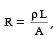ρ is constant for a material. Given that cross-sectional area varies. Therefore resistance varies.
• From ohm’s law V = IR as I constant and R varies, therefore V varies and corresponding electric field will also vary.
• Draft velocity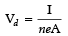will also vary as I constant and A is changed.Hence option (D) is correct.

Q.35: Three resistors having values R1, R2 and R3 are connected in series to a battery. Suppose R1 carries a current of 2.0 A, R2 has a resistance of 3.0 ohms and R3 dissipates 6.0 watts of power. Then the voltage across R3 is:
(a) 1 V
(b) 2 V
(c) 3 V
(d) 4 V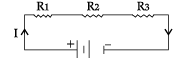Given I = 2 A, R2 = 3 W, P3 = 6 W
Power across R3 = V3I
6 = I2R3
6/4  = R= 3/2 = 1.5 Ω
V3 = IR3 = 2 (1.5) = 3 V

Q.36: A straight line plot showing the terminal potential difference (V) of a cell as a function of current (I) drawn from it, is shown in the figure. The internal resistance of the cell would be then: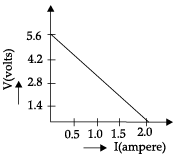(a) 2.8 ohms
(b) 1.4 ohms
(c) 1.2 ohms
(d) zero

I = O, V = E, ∴ E = 5.6 V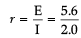= 2.8Ω

Q.37: A 10 m long wire of uniform cross-section and 20 Ω resistance is used in a potentiometer. The wire is connected in series with a battery of 5 V along with an external resistance of 480 Ω. If an unknown emf E is balanced at 6.0 m length of the wire, then the value of unknown emf is: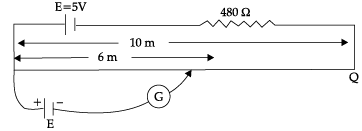(a) 1.2 V
(b) 1.02 V
(c) 0.2 V
(d) 0.12 V

Let PQ is a potentiometer wore of length 10 m,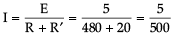= 1/100 = 0.01A
VPQ = I RPQ = 0.01 × 20
= 0.2 V
If 10 m potentiometer wire balances
⇒ 0.2 V
Then 1 m potentiometer wire balances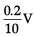Then 6 m potentiometer wire balances =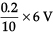=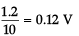Q.38: The current sensitivity of a galvanometer increases by 20%. If its resistance also increases by 25%, the voltage sensitivity will:
(a) decrease by 1%
(b) increased by 5%
(c) increased by 10%
(d) decrease by 4%

Given,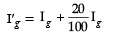=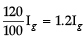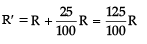= 1.25 R
V′g = ?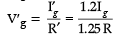=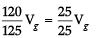% change =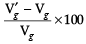=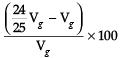=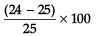=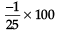= 4%
Decrease by 4%.

Q.39: Three infinitely long parallel straight current carrying wires A, B and C are kept at equal distance from each other as shown in the figure. The wire C experiences net force F. The net force on wire C, when the current in wire A is reversed will be:
(a) Zero
(b) F/2
(c) F
(d) 2F

Let F1 is force per unit, length between A & C
∴  i.e.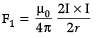And F2 is force per unit, length between B & C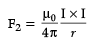Now net force on 'C' is per unit length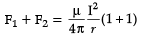=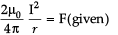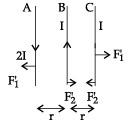Now
F′1 = Repulsive force between
A & C
=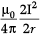F′2 = F2 = A reactive force between
B and C
∴ Net force on 'C' F′1 – F′2 = 0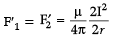∴ Net Force on 'C' is zero

Q.40: In a hydrogen atom, the electron moves in an orbit of radius 0.5 Ao making 10 revolutions per second, the magnetic moment associated with the orbital motion of the electron will be:
(a) 2.512 × 10–38 Am2
(b) 1.256 × 10–38Am2
(c) 0.628 × 10–38 Am2
(d) zero

R = 0.5 A°
ω = 10 rps = 10 × 2p rad/s
v = 10 Hz
M = IA = e v π r2
= 1.6 × 10–19 × 10 × 3.14 × 0.5 × 0.5 × 10–10 × 10–10
= 1.256 × 10–38 Am2

Q.41: An air-cored solenoid with length 30 cm, area of cross-section 25 cm2 and number of turns 800, carries a current of 2.5 A. The current is suddenly switched off in a brief time of 10–3 s. Ignoring the variation in magnetic field near the ends of the solenoid, the average back emf induced across the ends of the open switch in the circuit would be:
(a) zero
(b) 3.125 volts
(c) 6.54 volts
(d) 16.74 volts

Magnetic field inside a solenoid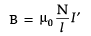Initial flux ∅1 = NBA =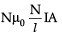=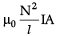=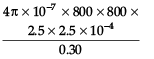= 16.74 × 10–3 Wb
Final flux ∅2 = 0 Average back emf |e|
=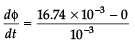= 16.74 V

Q.42: A sinusoidal voltage of peak value 283 V and frequency 50 Hz is applied to a series LCR circuit in which R = 3 Ω, L = 25.48 mH and C = 796 µF, then the power dissipated at the resonant condition will be:
(a) 39.70 kW
(b) 26.70 kW
(c) 13.35 kW
(d) Zero

Vo = 283 V, f = 50 Hz
R = 3 Ω, L = 25.48 mH
C = 796 µF
P|at resonance = ?
Power dissipated
P = I2R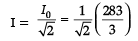= 66.7 A

P = I2R
= (66.7)23

= 13.35 kW

Q.43: A circular loop of radius 0.3 cm lies parallel to much bigger circular of radius 20 cm. The centre of the small loop is on the axis of the bigger loop. The distance between their centres is 15 cm. If a current of 2.0 A flows through the smaller loop, then the flux linked with the bigger loop is:
(a) 3.3 × 10–11 weber
(b) 6 × 10–11 weber
(c) 6.6 × 10–9 weber
(d) 9.1 × 10–11 weber

Let flux linked with smaller loop be ∅1 and with bigger loop be ∅2.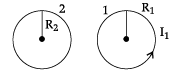Given R= 0.2 m
R1 = 0.003 m
x = 15 cm = 0.15 m
Now
1 = B2A1
=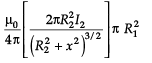M =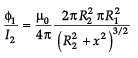Now ∅2 = M I1
=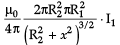= 9.1 × 10–11 Weber

Q.44: If both the number of turns and core length of an inductor is doubled keeping other factors constant, then its self-inductance will be:
(a) unaffected
(b) doubled
(c) halved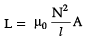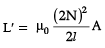=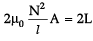Q.45: Given below are two statements labelled as Assertion (A) and Reason (R)
Assertion (A): To increase the range of an ammeter, we must connect a suitable high resistance in series to it.
Reason (R): The ammeter with increased range should have high resistance.
Select the most appropriate answer from the options given below:
(a) Both A and R are true and R is the correct explanation of A.
(b) Both A and R are true but R is not the correct explanation of A.
(c) A is true but R is false.
(d) A is false and R is also false
.

Correct option is (D) as both statements are false. To increase the range of an ammeter, suitable low R (or shunt) should be connected in parallel to it. The ammeter with increased range has low resistance.

Q.46: Given below are two statements labelled as Assertion (A) and Reason (R)
Assertion (A): An electron has a high potential energy when it is at a location associated with a more negative value of potential and a low potential energy when at a location associated with a more positive potential.
Reason (R): Electrons move from a region of higher potential to region of lower potential.
Select the most appropriate answer from the options given below:
(a) Both A and R are true and R is the correct explanation of A.
(b) Both A and R are true but R is not the correct explanation of A.
(c) A is true but R is false.
(d) A is false and R is also false.

Statements correct but reason is wrong because electrons move from a region of low potential to high potential.

Q.47: Given below are two statements labelled as Assertion (A) and Reason (R)
Assertion (A): A magnetic needle free to rotate in a vertical plane, orients itself (with its axis) vertical at the poles of the earth.
Reason (R): At the poles of the earth, the horizontal component of earth’s magnetic field will be zero.
Select the most appropriate answer from the options given below:
(a) Both A and R are true and R is the correct explanation of A.
(b) Both A and R are true but R is not the correct explanation of A.
(c) A is true but R is false.
(d) A is false and R is also false.

The given statement is correct and reason is the correct explanation of the above statement. At poles, magnetic needle orients itself vertically because horizontal components of earth’s field is zero there.

Q.48: Given below are two statements labelled as Assertion (A) and Reason (R)
Assertion (A): A proton and an electron, with same momenta, enter in a magnetic field in a direction at right angles to the lines of the force. The radius of the paths followed by them will be same.
Reason (R): Electron has less mass than the proton.
Select the most appropriate answer from the options given below:
(a) Both A and R are true and R is the correct explanation of A.
(b) Both A and R are true but R is not the correct explanation of A.
(c) A is true but R is false.
(d) A is false and R is also false.

we know,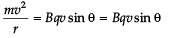Centripetal force = magnetic Lorentz force
sin θ = sin 90° = 1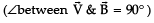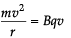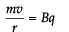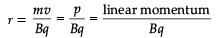Since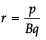Given p, B are same
Also q for proton & electron is same except its sign.
∴ Radius is same. So statement is correct but reason is not the correct explanation of the given assertion.

Q.49: Given below are two statements labelled as Assertion (A) and Reason (R)
Assertion (A): On Increasing the current sensitivity of a galvanometer by increasing the number of turns, may not necessarily increase its voltage sensitivity.
Reason (R): The resistance of the coil of the galvanometer increases on increasing the number of turns.
Select the most appropriate answer from the options given below:
(a) Both A and R are true and R is the correct explanation of A.
(b) Both A and R are true but R is not the correct explanation of A.
(c) A is true but R is false.
(d) A is false and R is also false.

When we increase current sensitivity by increasing number of turns, then resistance of coil also increases. So increasing current sensitivity does not necessarily imply that voltage sensitivity will increase because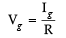∴ if Ig ↑ & R ↑ by different amounts, then Vg may increase or decrease.

Section - C
This section consists of 6 multiple choice questions with an overall choice to attempt any 5. In case more than desirable number of questions are attempted, ONLY first 5 will be considered for evaluation.

Q.50: A cylindrical bar magnet is rotated about its axis in the figure. A wire is connected from the axis and is made to touch the cylindrical surface through a contact. Then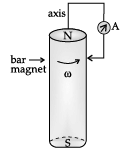(a) A direct current flows in the ammeter A.
(b) No current flows through the ammeter A.
(c) An alternating-sinusoidal current flows through the ammeter A with a time period 2p/w.
(d) A time varying non-sinusoidal current flows through the ammeter A.

The phenomenon of electromagnetic induction is used in this problem. Whenever the number of magnetic lines of force (magnetic flux) passing through a circuit change (or a moving conductor cuts the magnetic flux), an emf is produced in the circuit (or emf induces across the ends of the conductor) is called induced emf. The induced emf persists only as long as there is a change or cutting of flux.
When cylindrical bar magnet is rotated about its axis, no change in flux linked with the circuit takes place,  consequently no emf induces and hence, no current flows through the ammeter A. Hence the ammeter shows no deflection.

Q.51: There are two coils A and B as shown in figure. A current start flowing in B as shown, when A is moved towards B and stops when A stops moving. The current in A is counter clockwise. B is kept stationary when A moves. We can infer that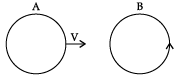(a) there is a constant current in the clockwise direction in A.
(b) there is a varying current in A.
(c) there is no current in A.
(d) there is a constant current in the counter clockwise direction in A.

When coil A moves towards coil B with constant velocity, so rate of change of magnetic flux due to coil B in coil A will be constant that gives constant current in coil A in same direction as in coil B by Lenz’s law.

CASE STUDY

Kirchhoff’s circuit laws: Kirchhoff’s laws were eventually named after Gustav Robert Kirchhoff and are now known as Kirchhoff’s current and Voltage Laws. Kirchhoff’s current states that at the junction of the circuit, the algebraic sum of the current meeting at any node in the circuit is zero, i.e., ∑ i = 0. Kirchhoff’s voltage states that the sum of every voltage drop in a loop for any closed network of a circuit is zero. This means while adding the voltage across each circuit element in any closed loop of circuit is zero. The figure illustrates that the total voltage around a closed loop must be zero.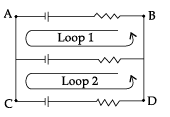The steps of solving circuit using Kirchhoff’s laws are as follows:

• The first and foremost step is to draw a closed loop to a circuit and draw the direction of the flow of current.
• Defining our sign convention is very important.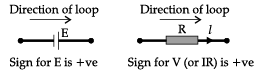• Defining our sign convention is very important.
• Apply Kirchhoff’s current law
• Apply Kirchhoff’s voltage law.

Q.52: Which law is followed to analyse the electric circuit?
(a) Newton’s law

(b) Coulomb’s law
(c) Kirchhoff ’s law
(d) Gravitational law

Apply Kirchhoff’s current junction law at point-b

(+I1)+(–I2)+(+I3) =0

I1–I2+I3 =0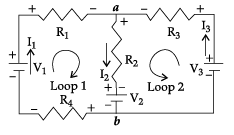Q.53: In the given figure, the relation between the currents at point b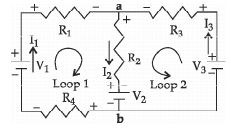(a) I1 −I2− I3 = 0
(b) I1 +I2 − I3 = 0
c) I1 −I2+ I3 = 0
(d) − I1+I2 − I3 = 0

According to the Kirchhoff’s current law, sum of the currents entering the junction is equal to the sum of the currents leaving the junction. Therefore, at node B Entering current = leaving current
I2 = I1 + I3
⇒ -I1 + I2 - I3 = 0

Q.54: On applying the Kirchhoff’s voltage law in loop 1, the obtained relationship is
(a) V+ I1R1 + I2R− V2 + I1R= 0
(b) V1 − I1R1 − I2R2 − V+ I1R4 = 0
(c) V1 − I1R1 − I2R− V2 − I1R= 0
(d) V1 + I1R1 + I2R− V2 − I1R4 = 0

According to the Kirchhoff’s second law, the sum of potential difference across the components is zero in any closed loop of a mash. Therefore, in loop 1, by following the sign conventional rule
V1 − I1R1 − I2R− V2 − I1R= 0

Q.55: On applying the Kirchhoff’s voltage law in loop 2, the obtained relationship is
(a) V3 − I3R3 − I2R2 − V2= 0
(b) V3 − I3R3 + I2R2 − V2= 0
(c) V3 + I3R3 + I2R2 − V2= 0
(d) V3 − I3R3 − I2R2 + V2= 0

Similar to the previous, by following the sign convention and applying the Kirchhoff’s voltage law in loop 2,
V3 − I3R3 − I2R2 − V2= 0

The document Class 12 Physics: CBSE Sample Question Paper- Term I (2021-22)- 3 Notes | Study Physics Class 12 - NEET is a part of the NEET Course Physics Class 12.
All you need of NEET at this link: NEETUse Code STAYHOME200 and get INR 200 additional OFF

## Physics Class 12

134 videos|388 docs|213 tests

### How to Prepare for NEET

Read our guide to prepare for NEET which is created by Toppers & the best Teachers

Track your progress, build streaks, highlight & save important lessons and more!

,

,

,

,

,

,

,

,

,

,

,

,

,

,

,

,

,

,

,

,

,

;